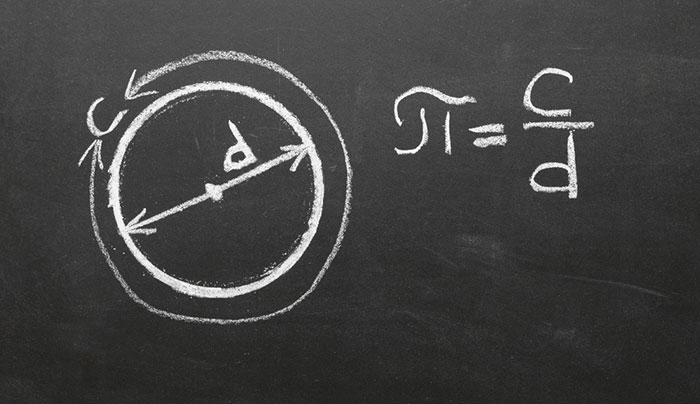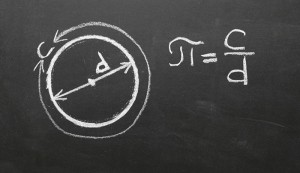/Happy Pi Day!## Happy Pi Day!Celebrate the day by visiting one of the fine eating establishments in San Marco for a Slice of Pi or some Pizza Pi.  🙂

Pi (π) is the ratio of a circle’s circumference to its diameter. Pi is a constant number, meaning that for all circles of any size, Pi will be the same.

The diameter of a circle is the distance from edge to edge, measuring straight through the center. The circumference of a circle is the distance around.

### HISTORY OF PI

By measuring circular objects, it has always turned out that a circle is a little more than 3 times its width around. In the Old Testament of the Bible (1 Kings 7:23), a circular pool is referred to as being 30 cubits around, and 10 cubits across. The mathematician Archimedes used polygons with many sides to approximate circles and determined that Pi was approximately 22/7. The symbol (Greek letter “π”) was first used in 1706 by William Jones. A ‘p’ was chosen for ‘perimeter’ of circles, and the use of π became popular after it was adopted by the Swiss mathematician Leonhard Euler in 1737. In recent years, Pi has been calculated to over one trillion digits past its decimal. Only 39 digits past the decimal are needed to accurately calculate the spherical volume of our entire universe, but because of Pi’s infinite & patternless nature, it’s a fun challenge to memorize, and to computationally calculate more and more digits.

3.14159265358979323846264338327950288419716939937510582097494459230781640628620899862803482534211706798214808651328230664709384460955058223172535940812848111745028410270193852110555964462294895493038196442881097566593344612847564823378678316527120190914564856692346034861045432664821339360726024914127372458700660631558817488152092096282925409171536436789259036001133053…

More at wikipedia!